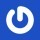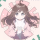## Description

1. 跳跃到其他摩天楼上；
2. 将消息传递给它当前所在的摩天楼上的其他 doge。

## Solution如图，蓝色的是虚点，5~9 是 0 的虚点，10~14 是 1 的虚点，25~29 是 4 的虚点，点 0 到点 1 的最短路为 5

k 如何取值？一般来说我们喜欢令 $k=\sqrt n$，但在这道题里却不是最优的，所以这道题有 $k=min\{100,\sqrt n\}$ 这种操作（$k$ 过大层数多反而没有直接暴力建边快）。

## Code

#include<bits/stdc++.h>
using namespace std;
int ret=0,f=1;char ch=getchar();
while (ch<'0'||ch>'9') {if (ch=='-') f=-f;ch=getchar();}
while (ch>='0'&&ch<='9') ret=ret*10+ch-'0',ch=getchar();
return ret*f;
}
int n,m;
bool v;
struct node{
int v,w,next;
}s;
s[top].v=b;
s[top].w=c;
}
void spfa(int x,int maxn){
queue<int> q;
for(int i=0;i<=maxn;i++){
d[i]=214748364;
v[i]=false;
}
d[x]=0;
q.push(x);
while(!q.empty()){
int now=q.front();
q.pop();
v[now]=false;
int to=s[i].v;
if(d[to]==214748364||d[to]>d[now]+s[i].w){
d[to]=d[now]+s[i].w;
if(!v[to]){
v[to]=true;
q.push(to);
}
}
}
}
}
int main(){
cin>>n>>m;
int siz=min(int(sqrt(n)),100);
for (int i=1;i<=siz;i++){
for (int j=0;j<=n-1;j++){
if (i+j>=n){
break;
}
}
for (int j=0;j<=n-1;j++){
}
}
int S,T;
for (int i=1;i<=m;i++){
if (i==1){
S=x;
}
if (i==2){
T=x;
}
if (y>siz){
for (int j=x-y,k=1;j>=1;j-=y,k++){
}
for (int j=x+y,k=1;j<=n-1;j+=y,k++){
}
}else{
}
}
spfa(S,(siz+1)*n);
if (d[T]>=214748364){
d[T]=-1;
}
cout<<d[T];
return 0;
}

## 评论

1.卓卓
Windows Chrome
5月前
2020-9-04 21:50:29

这个代码块是怎么做的？

•solstice23 博主
Android Chrome
5月前
2020-9-05 12:08:44

直接插入代码块，再在 Argon 设置里开启 Highlight.js 选项

## 发送评论编辑评论

|´・ω・)ノ
ヾ(≧∇≦*)ゝ
(☆ω☆)
（╯‵□′）╯︵┴─┴
￣﹃￣
(/ω＼)
∠( ᐛ 」∠)＿
(๑•̀ㅁ•́ฅ)
→_→
୧(๑•̀⌄•́๑)૭
٩(ˊᗜˋ*)و
(ノ°ο°)ノ
(´இ皿இ｀)
⌇●﹏●⌇
(ฅ´ω`ฅ)
(╯°A°)╯︵○○○
φ(￣∇￣o)
ヾ(´･ ･｀｡)ノ"
( ง ᵒ̌皿ᵒ̌)ง⁼³₌₃
(ó﹏ò｡)
Σ(っ °Д °;)っ
( ,,´･ω･)ﾉ"(´っω･｀｡)
╮(╯▽╰)╭
o(*////▽////*)q
＞﹏＜
( ๑´•ω•) "(ㆆᴗㆆ)

Emoji
AmashiroNatsukiEars